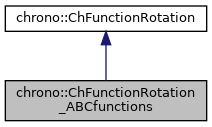chrono::ChFunctionRotation_ABCfunctions Class Reference

## Description

A rotation function q=f(s) where q(s) is defined with three ChFunction objects, each per an an angle in an intrinsic triplets of angles (ex.

Eulero angles, Cardano angles, etc), ex. A=A(s), B=B(s), C=C(s). By default, rotation is constant zero rotation. By default, the angleset is AngleSet::RXYZ (sequence: X-Y'-Z'' intrinsic), may be changed.

#include <ChFunctionRotation_ABCfunctions.h>

Inheritance diagram for chrono::ChFunctionRotation_ABCfunctions:[legend]
Collaboration diagram for chrono::ChFunctionRotation_ABCfunctions:[legend]

## Public Member Functions

ChFunctionRotation_ABCfunctions (const ChFunctionRotation_ABCfunctions &other)

virtual ChFunctionRotation_ABCfunctionsClone () const override
"Virtual" copy constructor.

void SetFunctionAngleA (std::shared_ptr< ChFunction > mx)
Set the angle function A=A(s) expressing the rotation about the first axis of rotation. Angle assumed in radians.

std::shared_ptr< ChFunctionGetFunctionAngleA () const
Get the angle function A=A(s) expressing the rotation about the fixed axis of rotation. Angle assumed in radians.

void SetFunctionAngleB (std::shared_ptr< ChFunction > mx)
Set the angle function B=B(s) expressing the rotation about the second axis of rotation. Angle assumed in radians.

std::shared_ptr< ChFunctionGetFunctionAngleB () const
Get the angle function B=B(s) expressing the rotation about the second axis of rotation. Angle assumed in radians.

void SetFunctionAngleC (std::shared_ptr< ChFunction > mx)
Set the angle function C=C(s) expressing the rotation about the second axis of rotation. Angle assumed in radians.

std::shared_ptr< ChFunctionGetFunctionAngleC () const
Get the angle function C=C(s) expressing the rotation about the second axis of rotation. Angle assumed in radians.

void SetAngleset (const AngleSet mset)
Set the angle set: Cardano, Eulero, etc. More...

AngleSet GetAngleset () const
Get the angle set: Cardano, Eulero, etc. The angle set define the order of the xyz axes of the intrinsic rotation.

virtual ChQuaternion Get_q (double s) const override
Return the rotation as a quaternion, function of s, as q=f(s).

virtual void ArchiveOUT (ChArchiveOut &marchive) override
Method to allow serialization of transient data to archives.

virtual void ArchiveIN (ChArchiveIn &marchive) override
Method to allow de-serialization of transient data from archives.Public Member Functions inherited from chrono::ChFunctionRotation
ChFunctionRotation (const ChFunctionRotation &other)

virtual ChVector Get_w_loc (double s) const
Return the derivative of the rotation function, at s, expressed as angular velocity w in local frame. More...

virtual ChVector Get_a_loc (double s) const
Return the derivative of the rotation function, at s, expressed as angular acceleration in local frame. More...

virtual void Estimate_s_domain (double &smin, double &smax) const
Return an estimate of the domain of the function argument. More...

virtual void Update (const double t)
Update could be implemented by children classes, ex. to launch callbacks.

## ◆ SetAngleset()

 void chrono::ChFunctionRotation_ABCfunctions::SetAngleset ( const AngleSet mset )
inline

Set the angle set: Cardano, Eulero, etc.

The angle set define the order of the xyz axes of the intrinsic rotation with the three A(s) B(s) C(s) rotations, for example Eulero angle set means that there is a rotation about Z, X', Z'', Cardano angle set means Z,X',Y', etc.

The documentation for this class was generated from the following files:
• /builds/uwsbel/chrono/src/chrono/motion_functions/ChFunctionRotation_ABCfunctions.h
• /builds/uwsbel/chrono/src/chrono/motion_functions/ChFunctionRotation_ABCfunctions.cpp